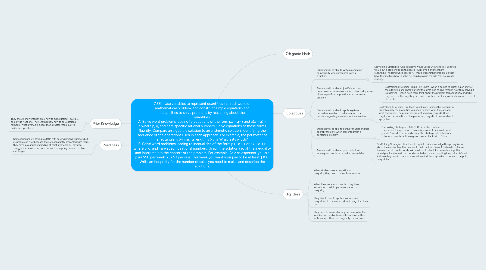# 7.EE.4 Use variables to represent quantities in a real-world or mathematical problem, and constru...

Get Started. It's Free7.EE.4 Use variables to represent quantities in a real-world or mathematical problem, and construct simple equations and inequalities to solve problems by reasoning about the quantities. A. Solve word problems leading to equations of the form px + q= r and p(x + q) = r, where p, q, and r are specific rational numbers. Solve equations of these forms fluently. Compare an algebraic solution to an arithmetic solution, identifying the sequence of the operations used in each approach. For example, the perimeter of a rectangle is 54 cm. Its length is 6 cm. What is its width? B. Solve word problems leading to inequalities of the form px +q > r or px + q < r, where p, q, and r are specific rational numbers. Graph the solution set of the inequality and interpret it in the context of the problem. For example: As a salesperson, you are paid \$50 per week plus \$3 per sale. This week you want your pay to be at least \$100. Write an inequality for the number of sales you need to make, and describe the solutions.## 2. Objectives

### 2.1. Students will be able to write an equation or inequality when presented with a situation.

2.1.1. Scaffolding Strategies- One strategy I would use is Show and Tell. So first I would put a problem up on the board. I will solve it and tell them everything I'm doing while solving it. I would put another problem up then have them tell me how to solve it. I could also use the I do, You do, We do strategy.

### 2.2. Students will be able to justify how they determined to write an equation or inequality when given a specific computational or contextual problem.

2.2.1. Scaffolding Strategies- Think-Pair-Share- When it comes to justifying an answer them thinking and speaking their thoughts, and talking through how they solved it is essential. I would have them think about the problem talk about how and why they solved it the way they did , and then share out their justifications with the rest of the class.

### 2.3. Students will be able to apply algebraic properties in order to find a solution to a problem regarding equations and inequalities.

2.3.1. Scaffolding Strategies- Pre teach vocabulary. I chose this because in order to apply the properties you need to know what they are and what they mean. The properties are just vocabulary words. Once they know the definition of the properties, they should know when to apply them.

### 2.4. Students will be able to define variables and use appropriate units when presented with a contextual problem.

2.4.1. Scaffolding Strategies- CUBES- C-circle the numbers. U-underline the question. B- box in key words. E- eliminate what you don't need. S-solve and check. This strategy helps students solve word problems. It also gives them a check list to follow.

### 2.5. Students will be able to graph solutions when given problems regarding inequalities.

2.5.1. Scaffolding Strategies- First I would tap into prior knowledge things they know about the number line, then we would build on that. Pause Ask Questions Pause Review- I would continuously ask questions to check for understanding. Then I would provide them with a checklist with the steps to graphing inequalities (Visual Aids) so they could know that they followed all the steps when it comes to graphing inequalities.